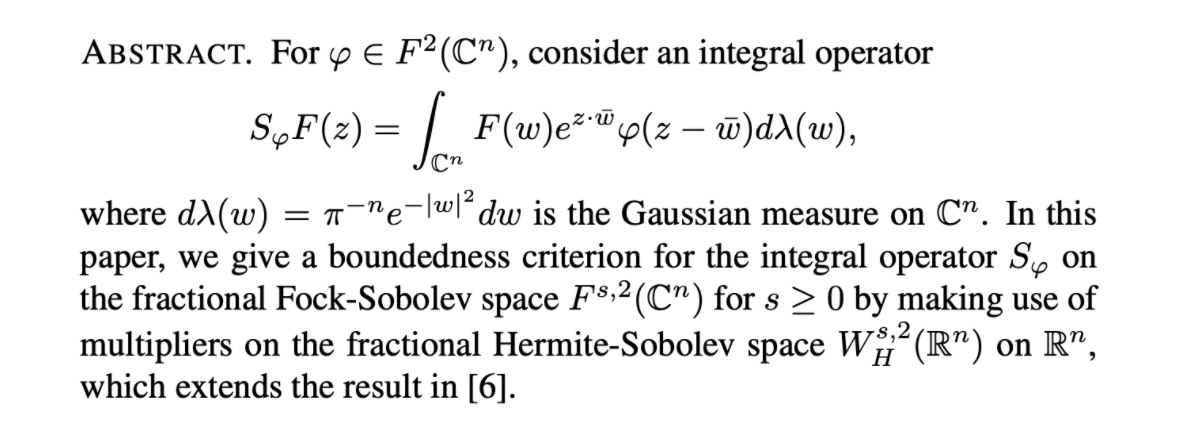﻿算子代数与算子理论工作会议-吉林大学数学学院

# 算子代数与算子理论工作会议

日  程 安 排

 11.1星期日 11.2星期一 11.3星期二 11.4星期三 11.5 星期四 11.6星期五 11.7星期六 9:30-10:20 9:30-10:20 9:30-10:10 9:30-10:10 9:30-10:10 9:30-10:10 9:30-10:20 ElliottGeorge LinHuaxin NiuZhuang NiuZhuang NiuZhuang 安庆楠 蒋春澜 10:30-11:10 10:30-11:10 10:20-11:00 10:20-11:00 10:20-11:00 10:20-11:00 NiuZhuang WangKun WangKun WangKun WangKun 刘智超 2:00-2:40 2:00-2:40 2:00-2:40 2:00-2:40 2:00-2:40 2:00-2:40 李春光 姚一隽 李建奎 侯绳照 曹广福 侯成军 2:45-3:25 2:45-3:25 2:45-3:25 2:45-3:25 2:45-3:25 2:45-3:25 崔博 王凯 武俊德 吴文明 王茂发 王利广 3:30-4:10 3:30-4:10 程国正 纪奎

2020年11月3日 星期二 上午  9:30-10:10;

2020年11月4日 星期三 上午  9:30-10:10;

2020年11月5日 星期四 上午  9:30-10:10.

2020年11月3日 星期二 上午10:20-11:00;

2020年11月4日 星期三 上午10:20-11:00;

2020年11月5日 星期四 上午10:20-11:00.

报告题目: Title: How many stars in the sky?---How many C*-algebras?

报告摘要: Abstract. When I first gave a talk with this title twenty-five years ago, it was on the occasion of receiving the CRM-Fields-PIMS Prize for beginning to answer this question. The purpose of the present lecture is to state the final answer---in the context of simple separable amenable C*-algebras, satisfying the UCT (perhaps automatic), and well-behaved in the sense of satisfying the equivalent criteria of the Toms-Winter conjecture.
     In  other words: "This many!"
    This answer summmarizes perhaps two thousand pages of work during well over fifty years (one might say, seventy-five years) by over a hundred contributors. It is perhaps close to being as imposing a body of work as that associated with the the Hubble and (its replacement) the James Webb space telescopes.

George A. Elliott教授: 是C*-代数分类理论的开创者,是国际算子代数及其应用领域的领袖人物.他提出了著名的“Elliott分类纲领”（也称为“Elliott猜想”）,数十年来引领和极大地推动了C*-代数理论的发展.1982年,George A. Elliott教授当选加拿大皇家科学院士;

1994年，被邀请在苏黎世国际数学家大会（ICM）上做报告； 1996年获得CRM-Fields Institute奖;1998年获得 Jeffery–Williams奖；1999年获得 John L. Synge Award奖；2008年，当选丹麦皇家科学院外籍院士；2012年，当选美国数学会首届会士。

of the corresponding crossed product $C^*$-algebra $C(X)\rtimes \mathbb{Z}^d$ is $1$. This gives a positive answer to a conjecture of Niu and Phillips. This talk is based on a joint work with Zhuang Niu.

We will report a unified classification for separable finite

simple amenable Jiang-Su stable C*-algebras in the UCT class.

These C*-algebras are classified by the Elliott invariant.

We will, in particular, discuss the Elliott invariant and a reduction

theorem.

Adv. Math.J. Funct. Anal.J. Noncommut. Geom.Math. Z.发表多篇论文。

In sharp contrast to the classical Bergman kernels, we show the existence of DPP's associated with infinite rank lacunary Bergman kernels which satisfy the Ghosh-Peres number rigidity. This is a joint work with prof. Yanqi Qiu

In this talk, we consider the classical Littlewood's theorem on Bergman spaces. In detail, let

$$(\mathcal{R}f)(z)=\sum_{n=0}^{\infty}\pm a_nz^n,\quad f(z)=\sum_{n=0}^{\infty}a_nz^n\in H(\mathbb{D}).$$

Firstly,   the space $\{f\in H(\mathbb{D}):\mathbb{P} {\mathcal{R}f\in L_a^p\}=1\}$ is characterized  entirely.  Moreover, we completely  determine the $pq$-graph of $\mathcal{R}: L_a^p\rightarrow L_a^q.$

J. Funct. Anal.,  TAMS 等国际顶尖SCI杂志发表论文十余篇。并获得多项国家自然科学基金项目资助。

In this talk,  we report some results on automatic continuity and answer a question of Essaleh and Peralta in Linear Algebra Appl.

538 (2018), 1–21.

This is a joint with Shan Li and Kaija Luo.

Fock空间上的任一有界线性算子可以表示为形如。本报告将介绍Fock空间上积分算子有界性研究方面的一些进展。特别是当是朱算子或系数乘子的情形。Motivated by classical result of linear fractional composition operators on Hardy spaces,  in this talk, we introduce that the linear combinations of two composition operators induced by linear symbols on the Hilbert space of Dirichlet series with square summable coefficients are compact only when each one of them is compact. Moreover, such rigid behavior holds partially for some more general symbols.

Point-line algebra is a concrete class of  sub-homogeneous algebras. Such algebra may have non-Riesz ordered K_0 group and arbitrary finite generated K_1group and quite a lot of interesting works are built by these algebras. We will list our resent results on the sub-homogeneous algebras，most of which are about KK-groups and classification.

One of the most fundamental questions one can ask in an operator algebra is: when are two operators unitarily equivalent? The present talk addresses the related problem of computing the distance d_U between unitary orbits in terms of spectral information. In 2008,  Elliott and Ciuperca defined two distances for any C*-algebra A that has stable rank one: one is d_U, which is defined between approximate unitary equivalence classes of *-homomorphisms from C_0(0,1] to A, and the other is d_W, defined on the semigroup of morphisms between the Cuntz semigroups, from Cu(C_0(0,1]) to Cu(A). (The distance d_W is based on the work of Weyl.) The above distances can also be regarded as  distances (pseudo-metrics) between positive elements. In this talk, we will show that the two distances are equal for C*-algebras with stable rank one and real rank zero.

《Math. Scand.》等国际学术期刊发表多篇科研论文

In this talk, we introduce notions of asymptotic continuous orbit equivalence and (strongly) asymptotic conjugacy for expansive systems, and characterize them in terms of the transformation groupoids, the principal groupoids coming from the local conjugacy relations and the semi-direct product groupoids of the principal groupoids by the canonical group actions, together with their associated reduced groupoid $C^*$-algebras. In particular, we show that two asymptotically essentially free expansive systems are  asymptotically continuous orbit equivalent if and only if the associated semi-direct product groupoids are topologically isomorphic if and only if there exists a $C^*$-algebra isomorphism preserving the canonical Cartan subalgebras between the corresponding reduced $C^*$-algebras of these semi-direct product groupoids.

Wigner’s theorem shows that every transition probability preserving surjection on the set of all rank one projections on a Hilbert space is induced by a unitary or an antiunitary. Wigner's theorem can be interpreted as a result of mappings that preserves certain metric on the set of projections. Recently, Geh\'{e}r and \u{S}emrl have characterized the general form of surjective isometries of the set of all projections on an infinite-dimensional separable Hilbert space using unitaries and antiunitaries. In this talk, we will study the surjective $L^2$-isometries of the projection lattice of an infinite dimensional Hilbert space and show that every such isometry can also be described by unitaries and antiunitaries. This talk is based on joint work with Wenming Wu and Wei Yuan.